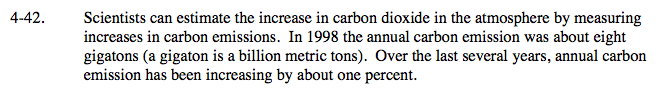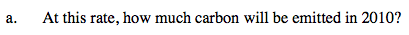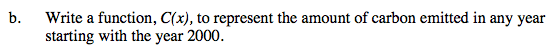### Home > A2C > Chapter 4 > Lesson 4.1.3 > Problem4-42

4-42.What multiplier will you use for something that is increasing by 1%?

9.015 gigatons$C(x)=(\text{beginning amount})(1.01)^{\text{time}}$

You can either calculate the begining amount for 2000 and let time = x or
you can let the beginning amount be the same as in 1998 and let time = x + 2.

C(x) = 8(1.01)(x + 2) or 8.16(1.01)x